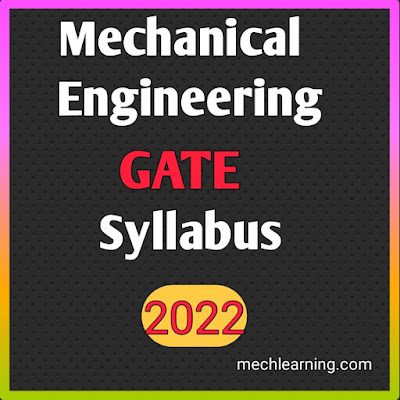# Mechanical engineering gate syllabus

## Graduate Aptitude Test in Engineering (GATE):-Mechanical engineering gate syllabus

## GATE Mechanical Engineering (ME) Exam syllabus 2022:-

The exam syllabus for mechanical engineering GATE 2022 is as follows:-

The overall GATE Mechanical Engineering (ME) Paper consists of 65 questions with a total of 100 marks with given 3 hours timing.

The syllabus divided into five (V) sections, each section consisting of different weightage of marks.

The detailed section wise syllabus for GATE 2022 mechanical engineering is as follows:-Mechanical engineering gate syllabus

### Section I  (13% of the total marks)

#### Engineering Mathematics:-

1. Linear Algebra:-

Matrix algebra, systems of the linear equations, eigen values and eigen vectors etc.

2. Calculus:-

Functions of variable (single), limits, continuity and differentiability, mean values theorems, indeterminate forms; evaluation of definite and improper integrals; integrals (double and triple),
partial derivatives equations, total derivative, Taylor series, maxima and minima,
Fourier series; gradient, divergence and curl, vector identities, directional derivatives, line integral, surface and volume integrals, Gauss, Stokes and Green’s theorems etc.

3. Differential equations:-

First order equations, higher order linear differential equations, Euler-Cauchy equation,
Laplace transforms; solutions of the heat equations, wave equations and Laplace equations etc.

4. Complex variables:-

Cauchy-Riemann equations, Cauchy’s integral theorem and integral formula, Taylors series.

5. Probability and Statistics:-

Definitions of the probability, conditional probability; mean, median, mode and standard deviation,
random variables, binomial distributions, Poisson distributions and normal distributions.

6. Numerical Methods:-

solutions of linear and non-linear equations, integration by trapezoidal and Simpson’s rules, single and multi-step methods for differential equations.

### Applied Mechanics and Design section:-

1. Engineering Mechanics:-

Free-body diagrams and equilibrium, friction of belt-pulley, brakes, clutches, etc,Trusses and frames,virtual work,
kinematics and dynamics of rigid bodies in plane motion, impulse and momentum and energy equations.

2. Strength of materials:-

Stress and strain, elastic constants, Poisson's ratio, Mohr’s circle for plane stress and plane strain,
thin cylinders; shear force and bending moment diagrams, strain gauges and rosettes,
bending and shear stresses, deflection of beams; torsion of circular shafts; Euler’s theory of columns; energy methods; thermal stresses;

3. Theory of Machines:-

Displacement, velocity and acceleration analysis of plane mechanisms, linkages, cams and followers,  balancing of reciprocating and rotating masses; gyroscope, gears and gear trains; flywheels and governors.

Vibrations:-

Free and forced vibration of single degree of freedom systems, effect of damping; vibration isolation, resonance, critical speeds of the shafts.

4. Machine Design:-

Design for static and dynamic loadings, failure of theories, fatigue strength and the S-N curves, rolling and sliding contact bearings, brakes and clutches, springs, bolted, riveted joints and welded joints; shafts, gears.

### Section III  (24% of the total marks)

#### Thermal engineering section:-

1. Fluid Mechanics:-

Properties of fluids, fluid statics, forces on submerged bodies, stability of floating bodies, Bernoulli’s equation; dimensional analysis, elementary turbulent flow, flow through pipes, head losses in pipes, bends and fittings; basics of compressible fluid flow, boundary layer Theory.

2. Heat-Transfer:-

Modes of heat transfer, one dimensional heat conduction, fins, unsteady heat conduction, heat exchanger performance, LMTD and NTU methods, radiative heat transfer, Stefan- Boltzmann law, Wien displacement law, black and grey surfaces, view factors, radiation network analysis.

3. Thermodynamics system:-

Thermodynamic systems and processes; properties of pure substances, behavior of ideal and real gases; zeroth and first laws of thermodynamics, work and heat, second law of thermodynamics; thermodynamic property charts and tables, availability and irreversibility; thermodynamic relations.

4. Applications:-

Power Engineering:-

Air and gas compressors; vapour cycle and gas turbine cycles, concepts of regeneration and reheat,

I.C. Engines:-

Air-standard cycle, Otto cycle, Diesel cycle and dual cycles.

Refrigeration and air conditioning:-

Vapour and gas refrigeration and heat pump cycles; properties of moist air, psychrometric chart, psychrometric processes.

Turbomachinery:-

Impulse and reaction principles, velocity diagrams, Pelton wheel, Francis Turbines and Kaplan turbines, steam turbine and gas turbines.

### Section IV  (24% of the total marks)

#### Manufacturing, and Industrial Engineering Section:-

1. Engineering Material science:-

Structure and properties of engineering materials, phase diagrams, heat treatment process, stress-strain diagrams for various engineering materials.

2. Manufacturing process:-

Casting, weldings, hot and cold working processes; forging, rolling, extrusion, drawing and sheet metal operations, forming processes, powder metallurgy.
Mechanics of machining; single and multi-point cutting tools, tool geometry and materials, tool life and wear; economics of machining; principles of nontraditional machining processes, jigs and fixtures, NC/CNC machines and CNC programming.

3. Computer Integrated Manufacturing

4. Operations Research:-

simplex method, PERT and CPM, assignment network model, Linear programming, network flow models, queuing models.

Forecasting models, aggregate production planning, scheduling, materials requirement planning; lean manufacturing, safety stock inventory control systems.

### Section V  (15% of the total marks)

#### General Aptitude (Common to all Branches)

1. Verbal Ability:-

English grammar; Sentence completion, Critical reasoning, Verbal reasoning.

2. Numerical Ability:-

Numerical computation; Numerical reasoning, Numerical estimation; Data interpretation (DI).

### GATE Mechanical Engineering (ME) Exam Patterns 2022:-

The detailed exam pattern for GATE 2022 is as follows:-

• Mode of Exam Online examination
• Duration of exam 3 hours
• Types of questions MCQs (multiple choice questions) and NAT (Non Answered Type)
• Sections main 3 sections – General Aptitude Section, Mathematics section and Subject-based section
• Total number of questions 65 questions
• Total marks 100 marks
• Negative Marking for exam For MCQs only.